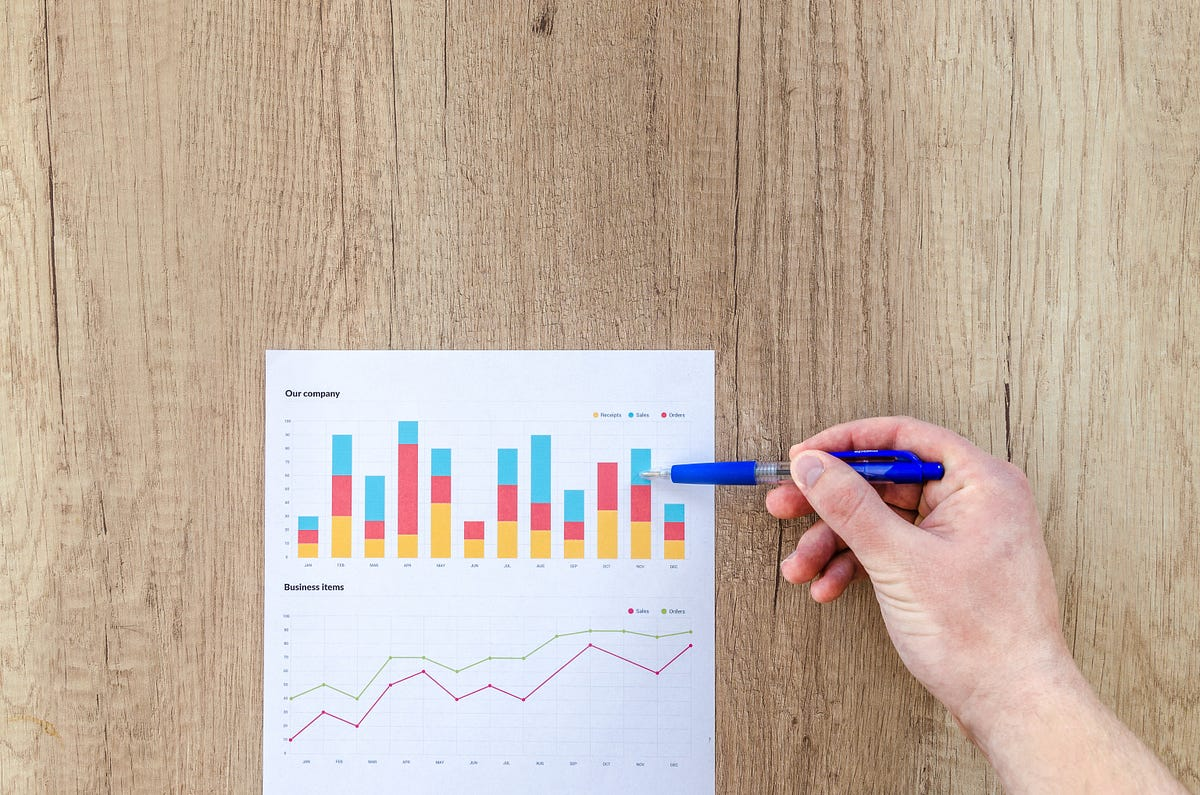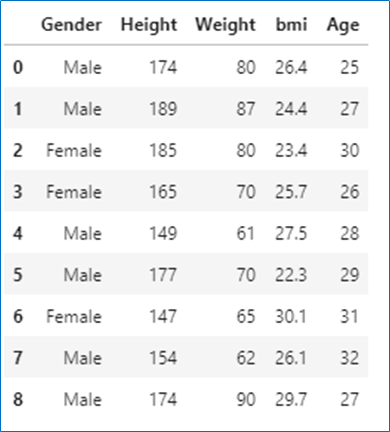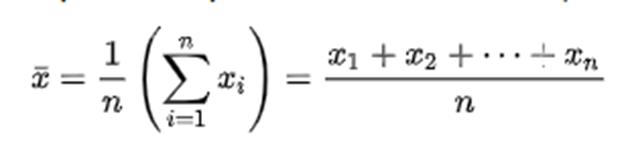# Exploring Descriptive Statistics Using Pandas and SeabornExploring Descriptive Statistics Using Pandas and Seaborn. Quantitative approach and Visual approach

## Descriptive Statistics in Python

Descriptive statistics include those that summarize the central tendency, dispersion, and shape of a dataset’s distribution.

1. Measure of central tendency
3. Measure of symmetry [ will save this for the future post]

## Dataset

Imported all the libraries needed for statistical plots and created a dataframe from the dataset given in `bmi.csv `file.

This dataset contains Height, Weight, Age, BMI, and Gender columns. Let’s calculate descriptive statistics for this dataset.

The code used in this project is available as a Jupyter Notebook on GitHub.

``````import numpy as np
import pandas as pd
import matplotlib.pyplot as plt
% matplotlib inline
df``````DataFrame

## Measure of Central Tendency

Measure of central tendency is used to describe the middle/center value of the data.

`Mean, Median, Mode` are measures of central tendency.

### 1. Mean

• Mean is the `average value` of the dataset.
• Mean is calculated by adding all values in the dataset divided by the number of values in the dataset.
• We can calculate the mean for only numerical variables

Formula to calculate mean## Applied Data Science with Python Certification Training Course -IgmGuru

Master Applied Data Science with Python and get noticed by the top Hiring Companies with IgmGuru's Data Science with Python Certification Program. Enroll Now

## Python for Data Science - Course for Beginners (Learn Python, Pandas)

This Python data science course will take you from knowing nothing about Python to coding and analyzing data with Python using tools like Pandas, NumPy, and ...

## Data Science Course in Dallas

Become a data analysis expert using the R programming language in this [data science](https://360digitmg.com/usa/data-science-using-python-and-r-programming-in-dallas "data science") certification training in Dallas, TX. You will master data...

## 50 Data Science Jobs That Opened Just Last Week

Data Science and Analytics market evolves to adapt to the constantly changing economic and business environments. Our latest survey report suggests that as the overall Data Science and Analytics market evolves to adapt to the constantly changing economic and business environments, data scientists and AI practitioners should be aware of the skills and tools that the broader community is working on. A good grip in these skills will further help data science enthusiasts to get the best jobs that various industries in their data science functions are offering.

## Python Programming & Data Handling

Python Programming & Data Handling